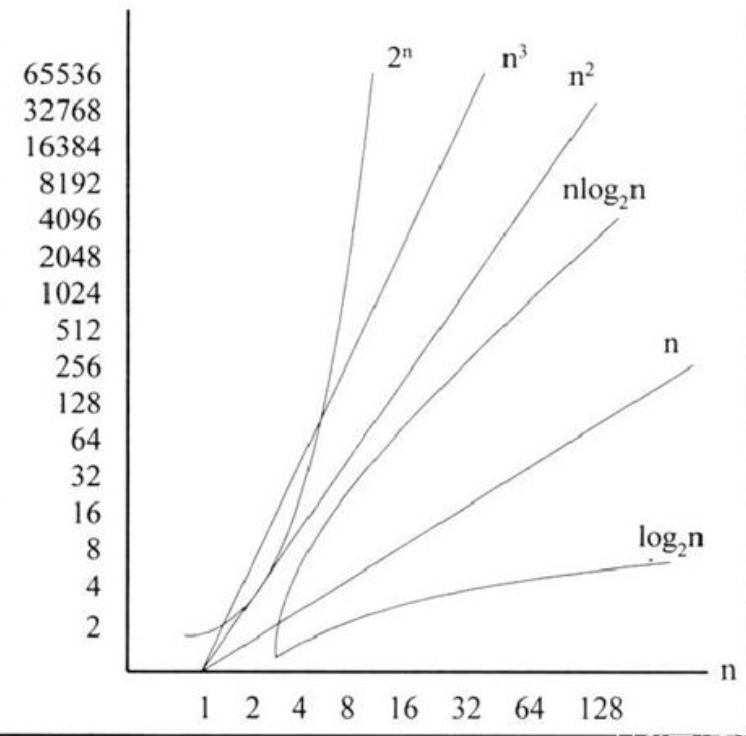## 1. 常数的时间复杂度O(1)

``````x = S
y = S
z = S
list = sort(x, y, z)
output list
``````

``````T(n) = O(3) + O(3) + O(1) = O(7) = O(1)
``````

## 2. 对数的时间复杂度O(logn)

``````23(10) = 212(3)
101(10) = 10202(3)
``````

``````while(n != 0)
n mod 3    // 取模
n = n/3    // 整除
``````

``````101 mod 3 = 2
n = 100/3 = 33
``````

``````33 mod 3 = 0
n = 33/3 = 11
``````

``````11 mod 3 = 2
n = 11/3 = 3
``````

``````3 mod 3 = 0
n = 3/3 = 1
``````

``````1 mod 3 = 1
n = 1/3 = 0
``````

``````O(2×(1+log3^n)) = O(log3n)
``````

## 3. 线性的时间复杂度O(n)

``````input(S))
s = 0
for a in S  // 遍历数据S中的元素a
s += a
output s
``````

``````O(1) + O(1)×n = O(n+1) = O(n)
``````

## 4. 平方的时间复杂度O(n^2)

``````input(S)
for (i=0; i>0; i--)       // 扫描次数，比较n个值
for (j=0; j<i; j++)   // 比较、交换次数
if S[j] > S[j+1]  // 比较，如果前面的数比后面的数大，则发生交换
temp = S[j]
S[j] = S[j+1]
S[j+1] = temp // 后面的数和前面的发生交换
output S
``````

``````(n-1) + (n-2) + (n-3) + ... + 3 + 2 + 1 = n(n-1)/2
``````

``````T(n) = O(n^2)
``````

## 5. 指数的时间复杂度O(2^n)

``````input(n)
power = 1
while (0 < n--)
power = power * 2
output power
``````

## 6. 算法复杂度总结``````c < log2^n < n < nlog2^n < n^2 < n^3 < 2^n < 3^n
``````